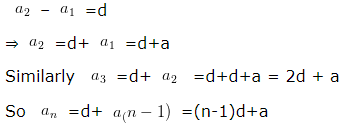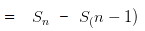# Arithmetic Progression Class 10 (AP) Maths

Arithmetic Progression Class 10 Maths, deals with various concepts which are as under:-

• Arithmetic Progressions
• nth Term of an AP
• Sum of First n Terms of an AP

### 1. Arithmetic Progression

#### Introduction of Arithmetic Progression, First term and Common Difference

Arithmetic Progression is a series of numbers, in which each successive termis obtained by adding a fixed number to the preceding term except the first term. that fixed term is known as common difference, which can be positive or negative.

### 2. nth term of an AP – Arithmetic Progression Class 10

If 1st term = a
Common difference = d
we know that for an AP### 3. Sum of n terms of an AP – Arithmetic Progression Class 10

a + (a + d) + (a + 2d) + (a + 3d)…………. + (a + (n – 1)d) = S ……………………. (1)
(a + (n – 1)d) + ……………………………+ (a + d) + a = S ……………………………….(2)
Add equation 1 and 2. We will get
2S = n{2a + (n – 1)d}
S = Sum of n terms of an AP = n/2{2a+(n-1)d}

### <span data-mce-type="bookmark" style="display: inline-block; width: 0px; overflow: hidden; line-height: 0;" class="mce_SELRES_start">﻿</span><span data-mce-type="bookmark" style="display: inline-block; width: 0px; overflow: hidden; line-height: 0;" class="mce_SELRES_start">﻿</span><span data-mce-type="bookmark" style="display: inline-block; width: 0px; overflow: hidden; line-height: 0;" class="mce_SELRES_start">﻿</span>

#### 1st and last term of Arithmetic Progression and Sum thereof

Sum of n terms of an AP = n/2 {2a+(n-1)d}=n/2 {a+a+(n-1)d}=n/2{a+an}
Because we know that nth term of AP = a + (n – 1)d

nth term of an AP = Difference between the sum of n terms and sum of (n – 1)terms